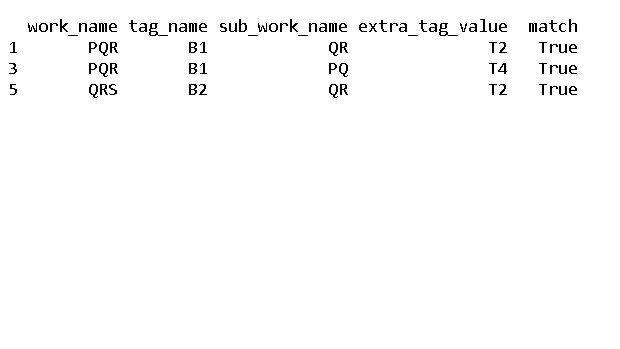Open In App
Related Articles
• Write an Interview Experience
• Pandas Exercises and Programs
• Different ways to create Pandas Dataframe

# Join Pandas DataFrames matching by substring

Prerequisites: Pandas

In this article, we will learn how to join two Data Frames matching by substring with python.

### Functions used:

• join(): joins all the elements in an iteration into a single string
• lambda(): an anonymous method which is declared without a name and can accept any number of parameters
• find(): gets the initial appearance of any requisite value
• merge(): merges two dataframes

### Approach

Follow the below steps to join two data frames matched by substring.

• Create two DataFrames.
• Join two dataframes using cartesian product
• Join a duplicate column including equal values in all the DataFrames
• Join the new column
• At last, remove the added column in each DataFrame.
• Then we need to add a new column to the Data frame. To do this we will use the “lambda” along with “find” functions where the output is greater than zero.
• Now we print the joined data frames matched by substrings .

Below is the implementation.

## Python3

 `import` `pandas as pd`` ` ` ` `dataFrame1 ``=` `pd.DataFrame([[``'PQR'``, ``'B1'``], [``'QRS'``, ``'B2'``], [``'RDE'``, ``'B3'``]], ``                          ``columns``=``[``'work_name'``, ``'tag_name'``])`` ` `dataFrame2 ``=` `pd.DataFrame([[``'RR'``, ``'T1'``], [``'QR'``, ``'T2'``], [``'HG'``, ``'T3'``], ``                           ``[``'PQ'``, ``'T4'``]],``                          ``columns``=``[``'sub_work_name'``, ``'extra_tag_value'``])`` ` `dataFrame1[``'join'``] ``=` `1``dataFrame2[``'join'``] ``=` `1`` ` `dataFrameFull ``=` `dataFrame1.merge(``  ``dataFrame2, on``=``'join'``).drop(``'join'``, axis``=``1``)`` ` `dataFrame2.drop(``'join'``, axis``=``1``, inplace``=``True``)`` ` `dataFrameFull[``'match'``] ``=` `dataFrameFull.``apply``(``    ``lambda` `x: x.work_name.find(x.sub_work_name), axis``=``1``).ge(``0``)`` ` `print``(dataFrameFull[dataFrameFull[``'match'``]])`

Output: